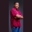Related Tags

swift
communitycreator

# How to create an octal value in Swift using the 0o keywordTheodore Kelechukwu Onyejiaku

### Overview

Numbers are present in the decimal format, like 10, 4, 235, and so on. However, there are other representations of number values. This includes binary representation, octal representation, hexadecimal representation, and so on. To create a variable and initialize it with an octal value, we use the 0o keyword.

### Syntax

0o(octalDigit)
The syntax to create octal value with 0o

### Parameters

0o: This is the keyword to create an octal value in Swift.

octalDigit: This is the octal digit we want to use.

### Return value

The value returned is an octal value.

### Example

// create variables with octal values
let octal1 = 0o4;
let octal2 = 0o7;
let octal3 = 0o0;
let octal4 = 0o75;

// print the decimal equivalents
print(octal1)
print(octal2)
print(octal3)
print(octal4)
Create octal values in Swift

### Explanation

• Lines 2-5: We create some variables and initialize them with some octal values.
• Lines 8-11: The values are printed to the console in decimal representations.

RELATED TAGS

swift
communitycreator

CONTRIBUTORTheodore Kelechukwu Onyejiaku
RELATED COURSES

View all Courses

Keep Exploring

Learn in-demand tech skills in half the time